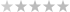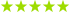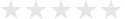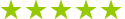# Calculus lessons in United States

## 120 calculus teachers in United States

0 teachers in my wish list

## 120 calculus teachers in United States(1 review)
Saber - Giza\$15
Math · Algebra · Calculus(3 reviews)
Math · Calculus(7 reviews)
Amaury - Paris\$29
Calculus(9 reviews)
Oliver - Barcelona\$60
Math · Economics for students · Calculus(4 reviews)
Thierry - Utrecht\$31
Math · Calculus · Economics for students(3 reviews)
Safiqul - Ongkharak\$17
Trusted teacher: I have a Doctorate degree in Mathematics. Maths is a wonderful subject, which one needs in all spheres. It is the basic of all subjects. A few applications are defined below: Trigonometry: Calculus is related with trigonometry and algebra. The fundamental trigonometric functions like sine and cosine are used to define the sound and light waves.Trigonometry is further used in oceanography to measure the heights of waves and tides in oceans. It is used in satellite systems, and to create maps. The famous Pythagoras' Theorem finds its' application in determining how tall a ladder needs to be in order to safely place the base away from the wall so it does not turn over. Probability and Statistics: Probability problems infer conclusions about characteristics of hypothetical data taken from the population. Probability explains the mathematical chance that something might happen, and is used in numerous day- to-day applications. Calculus : We can find the (or global) minimum and maximum values of a function as well as the relative (or local) minimum and maximum values of a function. Differential Equation: Differential equations are applied to predict the world around us, and used in a wide variety of disciplines, from biology, economics, physics, chemistry and engineering. It has applications in exponential growth and decay model, Newton's law of cooling, and Circuit theory, e.t.c. I have teaching experience for more than 15 years. My students are from all corners of the globe, and of all ages. I also make them learn Mathematics in English, so that they can excel in future. I am well acquainted will all the topics of mathematics, at all levels from schools to colleges. Children like my style of teaching.
Algebra · Calculus · Math(9 reviews)
Anmol - Enschede\$31
Math · Calculus · Statistics(11 reviews)
Tina - Ghent\$30
Math · Statistics · Calculus(1 review)
Randeep - Edmonton, AB\$33
Math · Algebra · Calculus(1 review)
Jude - Gothenburg\$28
Calculus · Math · Trigonometry(1 review)
Martin - Rome\$59
Calculus · Trigonometry · Statistics(1 review)
Ahmed - Cairo\$10
Math · Physics · Calculus(1 review)
Nahid - Amstelveen\$31
Math · Calculus · Algebra(3 reviews)
Eli - Helsinki\$36
Math · Algebra · Calculus
Showing results 1 - 25 of 3321 - 25 of 332

## Our students from United States evaluate their Calculus teacher.

### To ensure the quality of our Calculus teachers, we ask our students from United States to review them.Only reviews of students are published and they are guaranteed by Apprentus.Rated 4.9 out of 5 based on 55 reviews.

Math Tutoring: Algebra, Calculus, Probability Theory, Vector Calculus, Linear Algebra, Statistics, Trigonometry... (Enschede)
AnmolAnmol helped me with my statistics assignments and I am very happy with the level of support I received: He is very patient and repeats things when needed but also allows me to try my own approaches and give my own reasoning for the problems. He also provided me with a lot of additional material to further my learning. I highly recommend!
Review by FEDERICO
🚀EVERY CHILD IS A MATHS GENIUS | British Tutor 🇬🇧 (Toulouse)
OliverWe are just getting started, but our experiences with Oliver have been great so far. He is prompt to communicate, professional and very patient. He does a good job of understanding where my daughter is, helping her at her level, and not making her feel bad that she is having a tough time with Math. Highly recommend!
Review by TAMMY
Math,Calculus,Algebra,Geometry,Arithmetic,Trigonometry,Differential Equations,Mathematical Modeling,Numerical Analysis,Probability and Stats (Nakhon Nayok)
SafiqulMy son really enjoyed his first lesson with Safiqul. He told me that his brain felt 'super good' after the lesson. Just what my son needed - some challenge, kindness and patience. This is what Safiqul provided my son for his maths class. Thank very much and we hope to learn from you again very soon!
Review by ELLY